﻿ 基于正交拉丁方的混沌图像加密算法设计

# 基于正交拉丁方的混沌图像加密算法设计Design of Chaotic Image Encryption Algorithm Based on Orthogonal Latin Square

Abstract: This paper constructs an 8th-order orthogonal Latin square group and the algebraic formula of the reversible transformation determined by it, and gives the design method of the basic cryptographic system based on the orthogonal Latin square group. On this basis, a multiary stream cipher algorithm is designed in combination with the Henon chaotic system, the design method of  binary additive stream cipher is promoted, and the encryption effect of the new stream cipher algorithm on digital images is carried out by histogram, correlation, etc. Simulations show that the encryption algorithm has a good encryption effect.

1. 引言

1997年，Fridrich首次将混沌系统应用到数字图像加密中 。此后，因混沌系统具有初值敏感性和类随机性等特点，故在信息加密领域中得到广泛应用。由于一维混沌系统具有初始可控参数少，生成的序列随机性较差等缺点，因此，现在常用更高维的混沌系统来设计安全性更高的密码算法 。本文将先利用8阶正交拉丁方组设计一个基本系统，之后再结合二维Henon混沌系统所设计的密钥序列空间来完成整个流密码算法的设计。

2. Henon系统

Henon映射是一类二维非线性混沌系统，其映射方程  如下：

$\left\{\begin{array}{l}{x}_{n+1}=1-a{x}_{n}^{2}+{y}_{n}\hfill \\ {y}_{n+1}=b{x}_{n}\hfill \end{array}$ (1)

3. 基于正交拉丁方的流密码算法设计

3.1. 基本密码系统设计

${L}_{1}=\left[\begin{array}{cccccccc}7& 0& 1& 2& 3& 4& 5& 6\\ 6& 1& 0& 3& 2& 5& 4& 7\\ 5& 2& 3& 0& 1& 6& 7& 4\\ 4& 3& 2& 1& 0& 7& 6& 5\\ 3& 4& 5& 6& 7& 0& 1& 2\\ 2& 5& 4& 7& 6& 1& 0& 3\\ 1& 6& 7& 4& 5& 2& 3& 0\\ 0& 7& 6& 5& 4& 3& 2& 1\end{array}\right]=\left[\begin{array}{c}{T}_{0}\\ {T}_{1}\\ {T}_{2}\\ {T}_{3}\\ {T}_{4}\\ {T}_{5}\\ {T}_{6}\\ {T}_{7}\end{array}\right],\text{\hspace{0.17em}}\text{\hspace{0.17em}}{L}_{2}=\left[\begin{array}{cccccccc}7& 0& 1& 2& 3& 4& 5& 6\\ 3& 4& 5& 6& 7& 0& 1& 2\\ 6& 1& 0& 3& 2& 5& 4& 7\\ 2& 5& 4& 7& 6& 1& 0& 3\\ 1& 6& 7& 4& 5& 2& 3& 0\\ 5& 2& 3& 0& 1& 6& 7& 4\\ 0& 7& 6& 5& 4& 3& 2& 1\\ 4& 3& 2& 1& 0& 7& 6& 5\end{array}\right]=\left[\begin{array}{c}{T}_{8}\\ {T}_{9}\\ {T}_{10}\\ {T}_{11}\\ {T}_{12}\\ {T}_{13}\\ {T}_{14}\\ {T}_{15}\end{array}\right]$ (2)

${T}_{1}\left(0\right)=6,{T}_{1}\left(1\right)=1,{T}_{1}\left(2\right)=0,{T}_{1}\left(3\right)=3,{T}_{1}\left(4\right)=2,{T}_{1}\left(5\right)=5,{T}_{1}\left(6\right)=4,{T}_{1}\left(7\right)=7.$

$M=C={Z}_{8}={Z}_{2}^{3},\text{\hspace{0.17em}}\text{\hspace{0.17em}}T=\left\{{T}_{0},{T}_{1},{T}_{2},\cdots ,{T}_{15}\right\}.$

${T}_{0}\left(m\right)=\left(m+7\right)\mathrm{mod}8$

${T}_{1}\left(m\right)=\left[\left(3×{m}_{2}{m}_{3}+1\right)\mathrm{mod}4+4×{m}_{1}+1+4×{\stackrel{¯}{m}}_{3}\right]\mathrm{mod}8$

${T}_{2}\left(m\right)=\left[\left({m}_{2}{m}_{3}+2\right)\mathrm{mod}4+4×{m}_{1}+3+4×{m}_{3}\right]\mathrm{mod}8$

${T}_{3}\left(m\right)=\left[\left(3×{m}_{2}{m}_{3}+3\right)\mathrm{mod}4+4×{m}_{1}+1\right]\mathrm{mod}8$

${T}_{4}\left(m\right)=\left({m}_{2}{m}_{3}+4×{\stackrel{¯}{m}}_{1}+7\right)\mathrm{mod}8$

${T}_{5}\left(m\right)=\left[\left(3×{m}_{2}{m}_{3}+1\right)\mathrm{mod}4+4×{\stackrel{¯}{m}}_{1}+1+4×{\stackrel{¯}{m}}_{3}\right]\mathrm{mod}8$

${T}_{6}\left(m\right)=\left[\left({m}_{2}{m}_{3}+2\right)\mathrm{mod}4+4×{\stackrel{¯}{m}}_{1}+3+4×{m}_{3}\right]\mathrm{mod}8$

${T}_{7}\left(m\right)=\left[\left(3×{m}_{2}{m}_{3}+3\right)\mathrm{mod}4+4×{\stackrel{¯}{m}}_{1}+1\right]\mathrm{mod}8$

${T}_{0}^{-1}\left(c\right)=\left(c+1\right)\mathrm{mod}8$

${{T}^{\prime }}_{1}\left(c\right)=\left(3×{c}_{2}{c}_{3}+1\right)\mathrm{mod}4+4×{c}_{1},\text{\hspace{0.17em}}{T}_{1}^{-1}\left(c\right)={{T}^{\prime }}_{1}\left[\left(c+3+4×{c}_{3}\right)\mathrm{mod}8\right]$

${{T}^{\prime }}_{2}\left(c\right)=\left({c}_{2}{c}_{3}+2\right)\mathrm{mod}4+4×{c}_{1},\text{\hspace{0.17em}}{T}_{2}^{-1}\left(c\right)={{T}^{\prime }}_{2}\left[\left(c+1+4×{c}_{3}\right)\mathrm{mod}8\right]$

${{T}^{\prime }}_{3}\left(c\right)=\left(3×{c}_{2}{c}_{3}+3\right)\mathrm{mod}4+4×{c}_{1},\text{\hspace{0.17em}}{T}_{3}^{-1}\left(c\right)={{T}^{\prime }}_{3}\left[\left(c+7\right)\mathrm{mod}8\right]$

${{T}^{\prime }}_{4}\left(c\right)=\left({c}_{2}{c}_{3}+4×{\stackrel{¯}{c}}_{1}\right),\text{\hspace{0.17em}}{T}_{4}^{-1}\left(c\right)={{T}^{\prime }}_{4}\left[\left(c+1\right)\mathrm{mod}8\right]$

${{T}^{\prime }}_{5}\left(c\right)=\left(3×{c}_{2}{c}_{3}+1\right)\mathrm{mod}4+4×{\stackrel{¯}{c}}_{1},\text{\hspace{0.17em}}{T}_{5}^{-1}\left(c\right)={{T}^{\prime }}_{5}\left[\left(c+3+4×{c}_{3}\right)\mathrm{mod}8\right]$

${{T}^{\prime }}_{6}\left(c\right)=\left({c}_{2}{c}_{3}+2\right)\mathrm{mod}4+4×{\stackrel{¯}{c}}_{1},\text{\hspace{0.17em}}{T}_{6}^{-1}\left(c\right)={{T}^{\prime }}_{6}\left[\left(c+1+4×{c}_{3}\right)\mathrm{mod}8\right]$

${{T}^{\prime }}_{7}\left(c\right)=\left(3×{c}_{2}{c}_{3}+3\right)\mathrm{mod}4+4×{\stackrel{¯}{c}}_{1},\text{\hspace{0.17em}}{T}_{7}^{-1}\left(c\right)={{T}^{\prime }}_{7}\left[\left(c+7\right)\mathrm{mod}8\right]$

a) 基本加密变换E：对任意3比特明文 $m={m}_{1}{m}_{2}{m}_{3}\in {Z}_{8}$ 和4比特密钥 $k={k}_{1}{k}_{2}{k}_{3}{k}_{4}\in {Z}_{16}$，其中 ${m}_{1},{m}_{2},{m}_{3},{k}_{1},{k}_{2},{k}_{3},{k}_{4}\in {Z}_{2}$，可利用如下统一代数式将加密变换 $c=E\left(m,k\right)$ 设计为：

$\begin{array}{c}c={\stackrel{˜}{k}}_{0}×{T}_{0}\left(m\right)+{\stackrel{˜}{k}}_{1}×{T}_{1}\left(m\right)+{\stackrel{˜}{k}}_{2}×{T}_{2}\left(m\right)+{\stackrel{˜}{k}}_{3}×{T}_{3}\left(m\right)+{\stackrel{˜}{k}}_{4}×{T}_{4}\left(m\right)+{\stackrel{˜}{k}}_{5}×{T}_{5}\left(m\right)\\ \text{\hspace{0.17em}}\text{\hspace{0.17em}}+{\stackrel{˜}{k}}_{6}×{T}_{6}\left(m\right)+{\stackrel{˜}{k}}_{7}×{T}_{7}\left(m\right)+{\stackrel{˜}{k}}_{8}×{T}_{8}\left(m\right)+{\stackrel{˜}{k}}_{9}×{T}_{9}\left(m\right)+{\stackrel{˜}{k}}_{10}×{T}_{10}\left(m\right)\\ \text{\hspace{0.17em}}\text{\hspace{0.17em}}+{\stackrel{˜}{k}}_{11}×{T}_{11}\left(m\right)+{\stackrel{˜}{k}}_{12}×{T}_{12}\left(m\right)+{\stackrel{˜}{k}}_{13}×{T}_{13}\left(m\right)+{\stackrel{˜}{k}}_{14}×{T}_{14}\left(m\right)+{\stackrel{˜}{k}}_{15}×{T}_{15}\left( m \right)\end{array}$

${\stackrel{˜}{k}}_{9}={k}_{1}\wedge {\stackrel{¯}{k}}_{2}\wedge {\stackrel{¯}{k}}_{3}\wedge {k}_{4}$${\stackrel{˜}{k}}_{10}={k}_{1}\wedge {\stackrel{¯}{k}}_{2}\wedge {k}_{3}\wedge {\stackrel{¯}{k}}_{4}$${\stackrel{˜}{k}}_{11}={k}_{1}\wedge {\stackrel{¯}{k}}_{2}\wedge {k}_{3}\wedge {k}_{4}$${\stackrel{˜}{k}}_{12}={k}_{1}\wedge {k}_{2}\wedge {\stackrel{¯}{k}}_{3}\wedge {\stackrel{¯}{k}}_{4}$${\stackrel{˜}{k}}_{13}={k}_{1}\wedge {k}_{2}\wedge {\stackrel{¯}{k}}_{3}\wedge {k}_{4}$${\stackrel{˜}{k}}_{14}={k}_{1}\wedge {k}_{2}\wedge {k}_{3}\wedge {\stackrel{¯}{k}}_{4}$${\stackrel{˜}{k}}_{15}={k}_{1}\wedge {k}_{2}\wedge {k}_{3}\wedge {k}_{4}$

b) 基本解密变换D：对任意3比特密文 $c={c}_{1}{c}_{2}{c}_{3}\in {Z}_{8}$ 和4比特密钥 $k={k}_{1}{k}_{2}{k}_{3}{k}_{4}\in {Z}_{16}$，其中

${c}_{1},{c}_{2},{c}_{3},{k}_{1},{k}_{2},{k}_{3},{k}_{4}\in {Z}_{2}$，可利用如下统一代数式将解密变换 $m=D\left(c,k\right)$ 设计为： $m=\underset{i=0}{\overset{15}{\sum }}{\stackrel{˜}{k}}_{i}×{T}_{i}^{-1}\left(c\right)$

3.2. 算法设计

a) 选取大小为 $M×N$ 的灰度图像P，并将其表示成数字矩阵 $I={\left({m}_{ij}\right)}_{M×N}$，其中 ${m}_{ij}\in {Z}_{256},i\in \left\{0,1,\cdots ,M-1\right\},j\in \left\{0,1,\cdots ,N-1\right\}$

b) 将矩阵I中每个像素值读取的8比特序列 $m={\stackrel{˜}{m}}_{1}{\stackrel{˜}{m}}_{2}{\stackrel{˜}{m}}_{3}\cdots$ 转换为2元序列，其中， ${\stackrel{˜}{m}}_{1}={m}_{1}{m}_{2}{m}_{3}\cdots {m}_{8}$ 等。同时，对每个8比特进行逐3比特的分组，转换为8元序列，其中， ${\stackrel{¯}{m}}_{1}={m}_{1}{m}_{2}{m}_{3}\in {Z}_{8},{\stackrel{¯}{m}}_{2}={m}_{4}{m}_{5}{m}_{6}\in {Z}_{8}$，剩余2比特 ${m}_{7}{m}_{8}$ 不参与分组和加密，等等；

c) 选定初始参数 ${x}_{0}=0.000001,{y}_{0}=0.000001,a=1.4,b=0.3$ 代入Henon混沌系统，迭代产生混沌序列并对1进行取模运算，然后通过取整变换为2元序列，再将2元序列变换为16元密钥流序列 $z={\stackrel{˜}{k}}_{1}{\stackrel{˜}{k}}_{2}\cdots$，其中， ${\stackrel{˜}{k}}_{1}={k}_{1}{k}_{2}{k}_{3}{k}_{4}$，等等；

d) 加密变换：依次对步骤(b)处理后所得到的8元序列进行加密。先对明文序列m中每个8比特 ${\stackrel{˜}{m}}_{j}$ 中的分组如 ${\stackrel{¯}{m}}_{1}{\stackrel{¯}{m}}_{2}$ 进行加密 ${\stackrel{¯}{c}}_{i}=E\left({\stackrel{¯}{m}}_{i},{\stackrel{˜}{k}}_{j}\right),i=1,2;j=1,2,\cdots$，可得到加密后的分组序列 ${\stackrel{¯}{c}}_{1}{\stackrel{¯}{c}}_{2}$，转换成6比特后拼接上未作加密的2比特 ${m}_{7}{m}_{8}$，从而得到8比特的密文分组 ${\stackrel{˜}{c}}_{j}$，对矩阵I中的所有像素值加密完成后即可得到密文图像c

e) 解密变换：依次对经过步骤(d)加密处理的每个密文序列c进行解密。同样只对8比特密文分组 ${\stackrel{˜}{c}}_{j}$ 决定的3比特序列 ${\stackrel{¯}{c}}_{1}{\stackrel{¯}{c}}_{2}$ 进行解密 ${\stackrel{¯}{m}}_{i}=D\left({\stackrel{¯}{c}}_{i},{\stackrel{˜}{k}}_{j}\right),i=1,2;j=1,2,\cdots$，可得到解密后的序列 ${\stackrel{¯}{m}}_{1}{\stackrel{¯}{m}}_{2}$，转换成6比特后拼接上未作加密的2比特 ${m}_{7}{m}_{8}$，从而得到8比特的明文序列m，全部解密完成即可得到明文图像P

3.3. 仿真结果及分析(a) 原图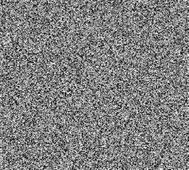(b) 本算法加密图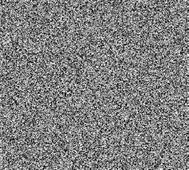(c) 对比算法加密图(d) 解密图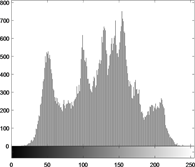(e) 原始图像直方图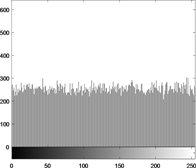(f) 本算法加密图像直方图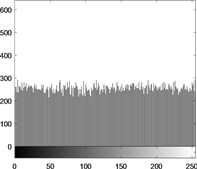(g) 对比算法加密图像直方图

Figure 1. Simulation effect of algorithm

3.3.1. 相关性分析(a)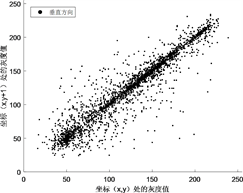(b)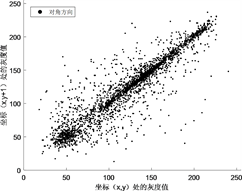(c)(d)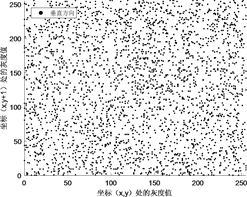(e)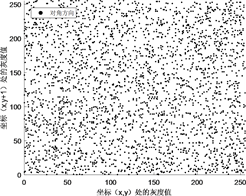(f)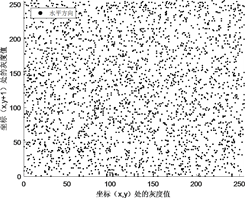(g)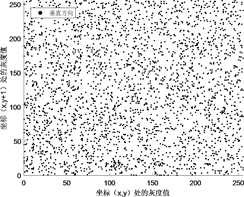(h)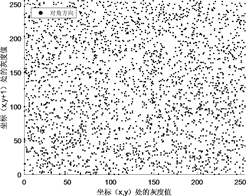(i)

Figure 2. Neighboring Pixel distribution

${\rho }_{xy}=\frac{\mathrm{cov}\left(x,y\right)}{\sqrt{D\left(x\right)}\sqrt{D\left(y\right)}}$ (3)

$\left\{\begin{array}{l}E\left(x\right)=\frac{1}{N}\underset{i=1}{\overset{N}{\sum }}{x}_{i}\\ D\left(x\right)=\frac{1}{N}\underset{i=1}{\overset{N}{\sum }}{\left({x}_{i}-E\left(x\right)\right)}^{2}\\ \mathrm{cov}\left(x,y\right)=\frac{1}{N}\underset{i=1}{\overset{N}{\sum }}\left({x}_{i}-E\left(x\right)\right)\left({y}_{i}-E\left(y\right)\right)\end{array}$ (4)Table 1. Image correlation coefficient

3.3.2. 密钥敏感度分析(a) 原图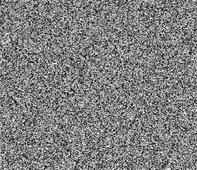(b) key1加密图(c) key1解密图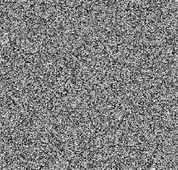(d) key2解密图

Figure 3. Encrypt and decrypt images with different keys

3.3.3. 信息熵分析

$H\left(m\right)=-\underset{i=0}{\overset{M-1}{\sum }}p\left({m}_{i}\right){\mathrm{log}}_{2}p\left({m}_{i}\right),\text{\hspace{0.17em}}\underset{i=0}{\overset{M-1}{\sum }}p\left({m}_{i}\right)=1$ (5)

4. 小结

 张斌, 徐超, 冯登国. 流密码的设计与分析: 回顾、现状与展望[J]. 密码学报, 2016, 3(6): 527-545.

 Shannon, C.E. (1949) Communication Theory of Secrecy System. Bell System Technical Journal, 28, 656-715.
https://doi.org/10.1002/j.1538-7305.1949.tb00928.x

 田传俊. 密钥非均匀分布的完善保密通信系统[J]. 通信学报, 2018, 39(11): 1-9.

 田传俊. 基于4阶正交拉丁方组实际基本密码系统设计[J]. 深圳大学学报(理工版), 2020, 37(3): 251-256.

 Fridrich, J. (1997) Image Encryption Based on Chaotic Maps. IEEE International Con-ference on Systems, Orlando, FL, 12-15 October 1997, 1105-1110.

 朱和贵, 蒲宝明, 朱志良, 赵怡然, 宋禹佳. 二维Sine-Tent超混沌映射及其在图像加密中的应用[J]. 小型微型计算机系统, 2019, 40(7): 1510-1518.

 Hénon, M. (1976) A Two-Dimensional Mapping with a Strange Attractor. Communications in Math-ematical Physics, 50, 69-77.
https://doi.org/10.1007/BF01608556

 缑新科, 吴贻峰. 基于复合混沌的数字图像加密算法[J]. 计算机与数字工程, 2018, 46(12): 2574-2579.

Top